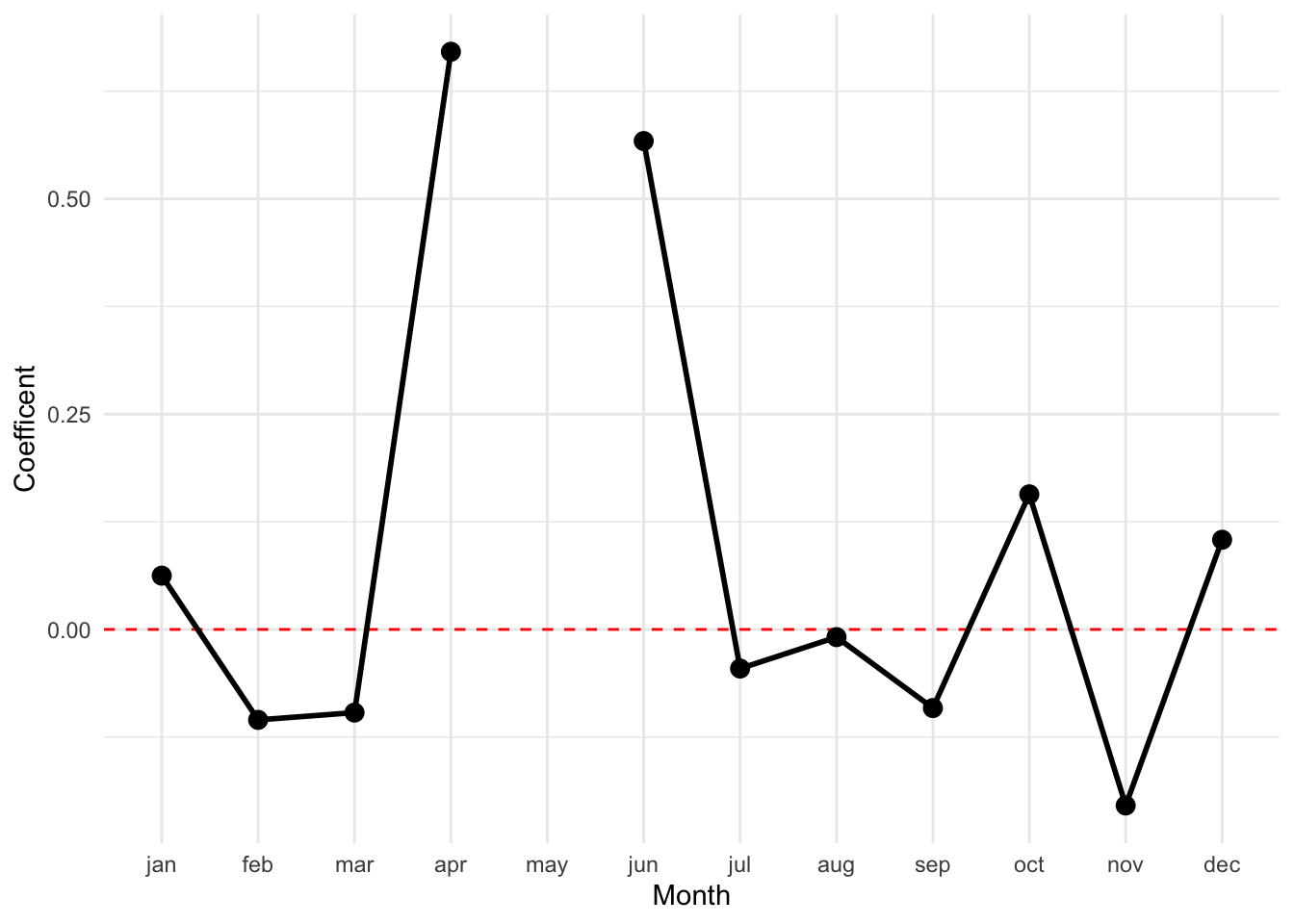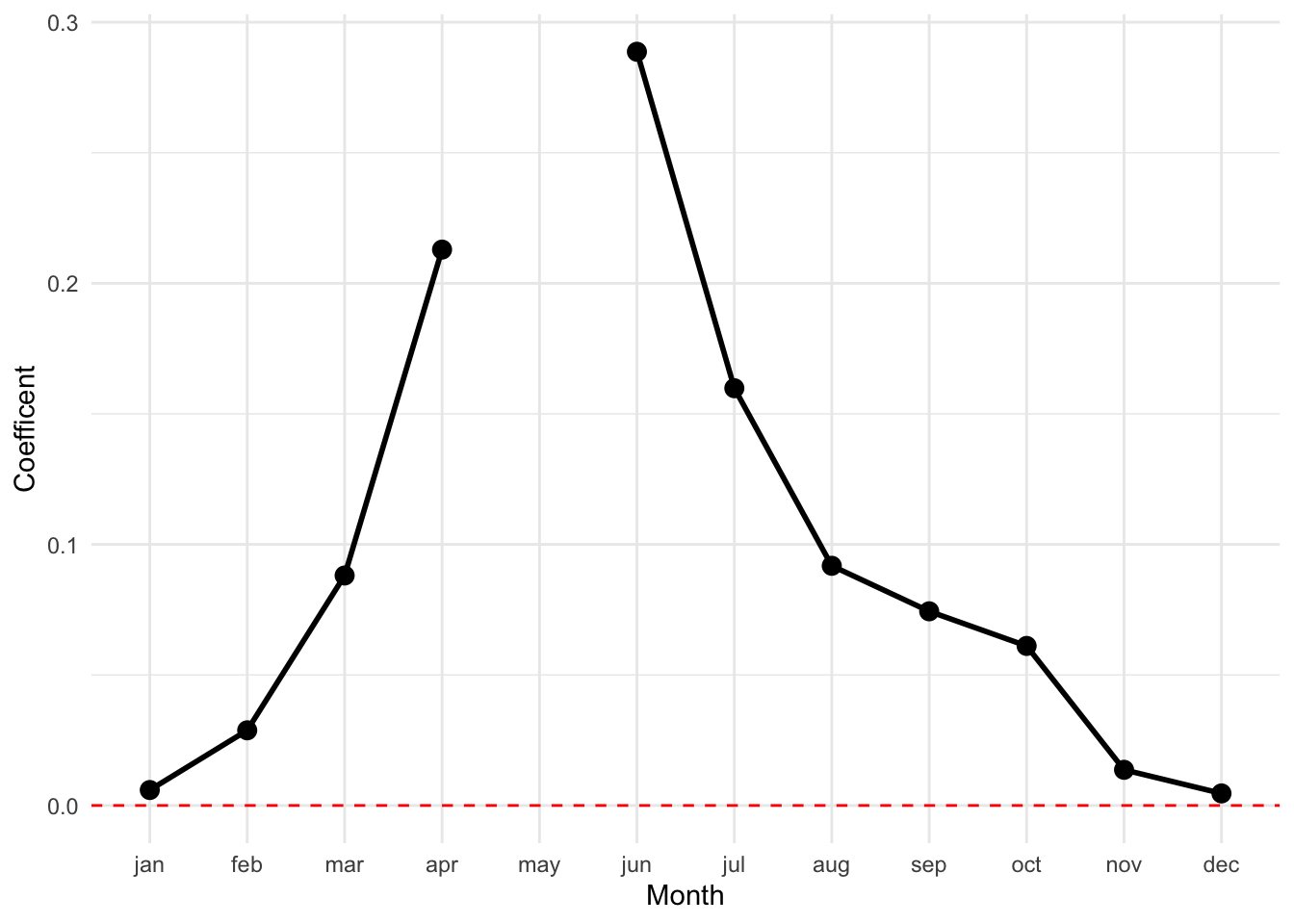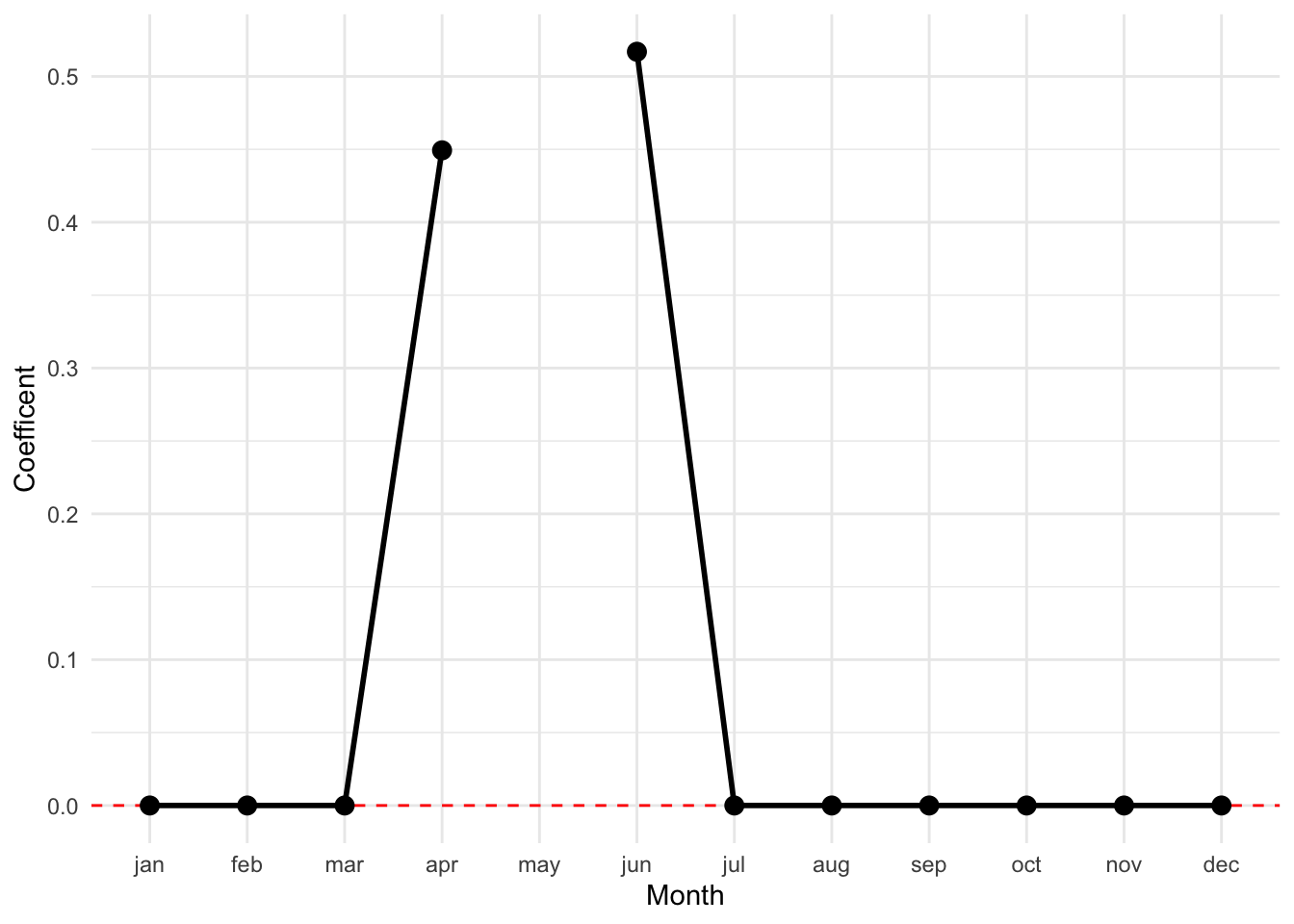library(tidyverse)
library(forcats)
library(ggrepel)
library(smodels)
library(glmnet)

theme_set(theme_minimal())
options(dplyr.summarise.inform = FALSE)
options(width = 77L)

## City Tempurature Data

Today we will start by looking at a small data set of temperatures from 327 cities across the world.

temps <- read_csv("data/city_temps_yr.csv")
temps
## # A tibble: 327 x 14
##      may country  city    jan   feb   mar   apr   jun   jul   aug   sep   oct
##    <dbl> <chr>    <chr> <dbl> <dbl> <dbl> <dbl> <dbl> <dbl> <dbl> <dbl> <dbl>
##  1  27   Ivory C… Gagn…  26    27    27    27    26    25    25    25    26
##  2  26   Ivory C… Boua…  27    28    28    27    25    24    24    24    25
##  3  27   Ivory C… Abid…  27    28    28    28    26    25    24    25    26
##  4  27   Ivory C… Odie…  25    27    28    28    26    25    25    25    25
##  5  27   Benin    Coto…  27    28    28    28    26    26    25    26    26
##  6  27   Benin    Para…  26    28    29    29    26    25    25    25    26
##  7  30   Benin    Kandi  25    27    31    32    28    26    26    26    27
##  8  27   Togo     Lomé   27    28    28    28    26    25    25    26    26
##  9  30   Togo     Mango  27    30    31    31    27    26    26    26    27
## 10  28.4 Ghana    Accra  28.8  29.4  29.6  29.5  26.8  26.3  25.9  26.8  26.2
## # … with 317 more rows, and 2 more variables: nov <dbl>, dec <dbl>

Our goal is to predict the average daily high temperature for the month of May using the average daily temperatures for the other eleven months of the year. This is not particularly hard but will be a great illustration of linear regression regularization.

### Ordinary regression

We can start by predicting the average daily high in May as a function of all of the other eleven months. We can understand these coefficients best by plotting them over the course of the year (I have hidden to code because it is only useful in this one example):As you might expect, April and Jun have the largest coefficients. You may not have thought that other months would have negative weights. What is going on here? These variables have fairly high correlations, so we can get a fairly predictive model by combining positive weights on some variables and negative weights on others. This is a less-extreme version of the thought experiment we openned with.

## Regularization

The tendency of linear regression to overfit the data in the presence of highly-correlated variables or sets of variables is a major issue in predictive modeling. So far our only option has been to control this by limiting the number of variables in a model, either directly or by limiting the number of interactions or basis expansion terms.

Regularization is a more direct approach to limiting the complexity of a model. In general, it works by optimizing the fit of the data (such as the sum of squared errors) plus the complexity of the model. A tuning parameter gives the balance between the two measurements:

$\text{FIT} + \lambda \times \text{COMPLEXITY}$

Different choices of how to measure the complexity of the linear regression yield different types of models, which we will now investigate.

### Ridge regression

Ridge regression sets the complexity to be the sum of the squared regression parameters. Specifically, we have the following equation to minimize:

$\sum_i \left( y_i - x_i^t b \right)^2 + \lambda \cdot \sum_j b_j^2$

If lambda is very large, the best model will have no coefficients. As lambda approaches zero, the ridge vector will limit towards the ordinary least squares solution.

We will see how to write the code to fit this model in a moment. Let’s just look at the output for now:Now, the weights are all positive and spread out over the course of the year. There is still, however, a peak near April and June. What has happened is two fold: the penalty as made it “not worth” making offsetting negative weights and positive weights even if this slightly decreases the RMSE. The penalty is simply too great. Secondly, the ridge penalty in particular prefers many small weights compared to a few larger weights. That is why the values are spread throughout the year.

### Lasso Regression

Lasso regression uses the sum of absolute values of the regression coefficents as a penalty:

$\sum_i \left( y_i - x_i^t b \right)^2 + \lambda \cdot \sum_j | b_j |$

The behavior of the lasso regression has a very special property that can be extremely useful in predictive modeling. It tends to put a weight of exactly zero on terms that do not seem particularly important to the model predictions:The model has made every weight other than April and June set to exactly zero. As with ridge regression, the penalty is too great to make canceling negative weights and postive weights. Unlike ridge regression, the lasso penalty does not prefer many small weights to fewer large weights. Therefore, the model will pick only those variables which are most strongly correlated with the response; here, these are April and June.

The magic of lasso regression is that it sets many terms exactly to zero. This is accomplished because the absolute value does not have a derivative at zero. Therefore, the target function has many critical points where beta coefficents are equal to zero and a non-zero chance of setting any beta value to zero.

Which model would the lasso regression prefer in our left leg prediction example? It would put all of the weight on one arm, whichever one had a slightly higher correlation with the response.

### Elastic Net

The elastic net refers to a collection of models that sit in-between ridge and lasso regression. It is defined as a weighted sum of the absolute value of the coefficients and the square of the coefficients.

$\sum_i \left( y_i - x_i^t b \right)^2 + \lambda \cdot \sum_j | b_j | \cdot (\alpha) + | b_j |^2 \cdot (1 - \alpha)$

Here is the fit for our data with alpha equal to 0.2: